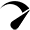# Lithium Battery Watt Hour Calculator: (mAh / Ah ↔ Wh)

Use our lithium (LiFePO4) battery watt-hour calculator to convert the battery capacity from amp hours (Ah), or milliamp hours (mAh) to watt hours (Wh).

Two columns
Vertical
Horizontal

## Usable Watt Hours(WH)

{{ field.label }} {{ field.converted }} {{ field.extraView }}
{{ option.label }} {{ option.converted }}
{{ item.label }} {{ item.data.converted }}

Issued on: {{ \$store.getters.getIssuedOn }}
Payment method: {{ \$store.getters.getPaymentType }}
{{ item.label }}: {{ item.converted }}

### How To Use This Calculator?

1 – Enter the battery capacity and select the unit type. The unit types are amp-hours (Ah), and milliamp-hours (mAh). For example, if you have a 100ah battery, enter 100 and select the unit type to Ah.

2 – Enter the battery voltage. Is this a 6v, 12v, 24v, or a 48v battery? It should be mentioned on the specs sheet of your battery or on the battery itself.

3 – Optional: Enter the number of batteries if you’re using multiple batteries. (Default value will be 1)

## How Do You Calculate Lithium Battery Watt-Hours?

Multiply the battery capacity in amp-hours (Ah) by the battery voltage to calculate watt hours (Wh).

Formula: Battery capacity Watt-hours = Battery capacity Ah × Battery voltage

### Example

Let’s say you have a 12v 200ah lithium battery.

``12v 200Ah battery into watt hours = 200 × 12 = 2400Wh``

## Lithium Battery Amp-Hours To Watt Hour Calculation

AdvertisementsHere’s a chart about different capacity (Ah) lithium batteries into watt hours @ 12v, 24, and 48v.

## Why Calculate Battery Watt-Hours?

Energy is equal to amp-hours multiplied by volts. Converting battery amp hours to watt-hours will give an idea of how much actual energy your battery can store or deliver.

For example, A 36v 12.8ah battery can store or deliver more energy than a 12v 30ah battery.

``36v * 12.8ah = 460.8Wh``
``12v * 30ah = 360Wh``

The benefit of converting battery amp hours to watt-hours:

• Makes it easy to calculate the battery runtime on a specific load. The energy or power consumption for most of the appliances is mentioned in watts or watt-hours. So, converting battery capacity in watt hours will make it easy for you to estimate the battery runtime on a load.
Scroll to TopOptimized by Seraphinite Accelerator
Turns on site high speed to be attractive for people and search engines.